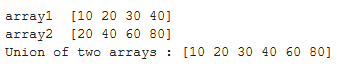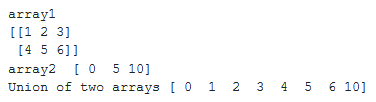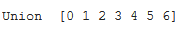Find the union of two NumPy arrays

• Last Updated : 05 Sep, 2020

To find union of two 1-dimensional arrays we can use function numpy.union1d() of Python Numpy library. It returns unique, sorted array with values that are in either of the two input arrays.

Syntax:

numpy.union1d(array1, array2)

Note The arrays given in input are flattened if they are not 1-dimensional.

Let’s see examples of how to find union of two arrays.

Example 1:

 # import librariesimport numpy as np    arr1 = np.array([10, 20, 30, 40])print("array1 ", arr1)  arr2 = np.array([20, 40, 60, 80])print("array2 ", arr2)  # print union of the two arraysprint("Union of two arrays :", np.union1d(arr1, arr2))

Output:Example 2:
Let’s see example of finding union of a 2-d and a 1-d array. As discussed earlier, if array passed as arguments to function numpy.union1d is 2-dimensional, then they are flattened to 1-dimension.

 # import librariesimport numpy as np    # 2-d arrayarr1 = np.array([[1, 2, 3], [4, 5, 6]])print("array1 ")print(arr1)  arr2 = np.array([0, 5, 10])print("array2 ", arr2)  # print union of 2-d array and 1-d arrayprint("Union of two arrays", np.union1d(arr1, arr2))

Output:Example3:
If we want to find union of more than two arrays, then we can find that by using functools.reduce function.

 # code to find union of more than two arrays# import librariesimport numpy as npfrom functools import reduce    array = reduce(np.union1d, ([1, 2, 3], [1, 3, 5],                            [2, 4, 6], [0, 0, 0]))print("Union ", array)

Output:My Personal Notes arrow_drop_up